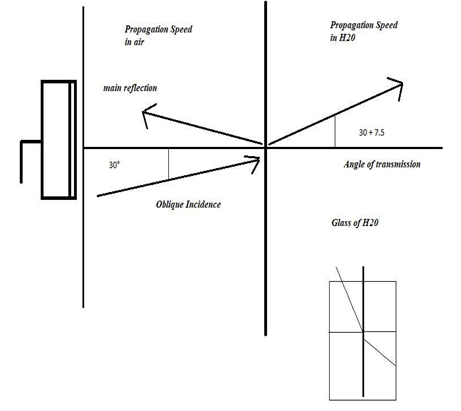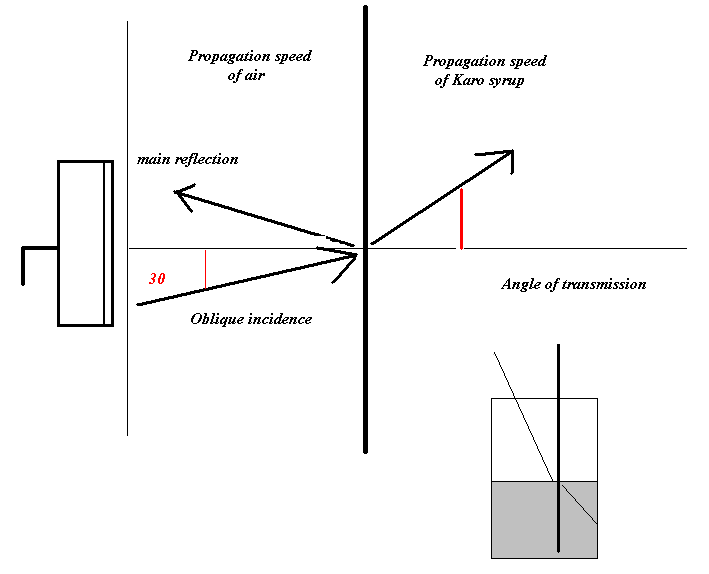# Physics of Ultrasound

Snell’s Law [in-class demonstration]

The concept that sound reflects and propagates in varied angles is an abstract concept that many students struggle to understand. I review this concept by providing an in-class demonstration that makes this less abstract and something that can be seen with glasses of liquids.If speed 1 < speed 2, then the incident angle < transmitted angle.

The difference in the stiffness and resulting propagation speeds helps to explain why the straw appears to be “broken” when you look through the side of the glass of water. The angle of transmission is measured against the vertical black line drawn on the glass of water. This helps to illustrate the 30-degree oblique incidence vs. the increased angle of transmission. A real-world example would be the change in imaging of a needle in a fluid-filled structure.

Example:

The propagation speed of sound through air is 900 m/sec while the propagation speed of sound through water is 1200 m/sec. To figure out the change in the angle of transmission, we form a ratio that will allow us to arrive at a percentage of change. So, 900/1200 = .75 and, therefore, that ratio of change from air to water in the glass is 100 – 75 = 25%. To figure out the angle, take 30 times .25 = 7.5 degrees. Therefore, 30 + 7.5 = 37.5 degree angle of transmission.

Now, consider a different glass of liquid as part of this demonstration by viewing a glass of Karo syrup.This time, the glass is filled with Karo syrup, which is stiffer and denser than the water, and the transmitted angle is greater due to the increased ability to travel quickly in the second media.

If speed 1 < speed 2, then the incident angle < transmitted angle.

Example:

The propagation speed of sound through air is 900 m/sec while the propagation speed of sound through Karo is 1500 m/sec. To figure out the change in the angle of transmission, we form a ratio that will allow us to arrive at a percentage of change. So, 900/1500 = .60 and, therefore, the ratio of change from air to Karo syrup in the glass is 100 – 60 = 40% gain. To figure out the angle, take 30 times .4 = 12 degrees. 30 + 12 = 42 degree angle of transmission. The real world example for this is noting a speed propagation artifact.

A final demonstration can be a glass that has 1/3 air, 1/3 vinegar, and 1/3 cooking oil. Do not forget to add a straw so that several bends in the straw are noted by viewing through the side of the glass.

Kevin D. Evans, PhD, RT (R) (M) (BD), RDMS, RVS, FSDMS, FAIUM, is Chair and Professor of Radiologic Sciences and Respiratory Therapy at The Ohio State University in Columbus, OH.